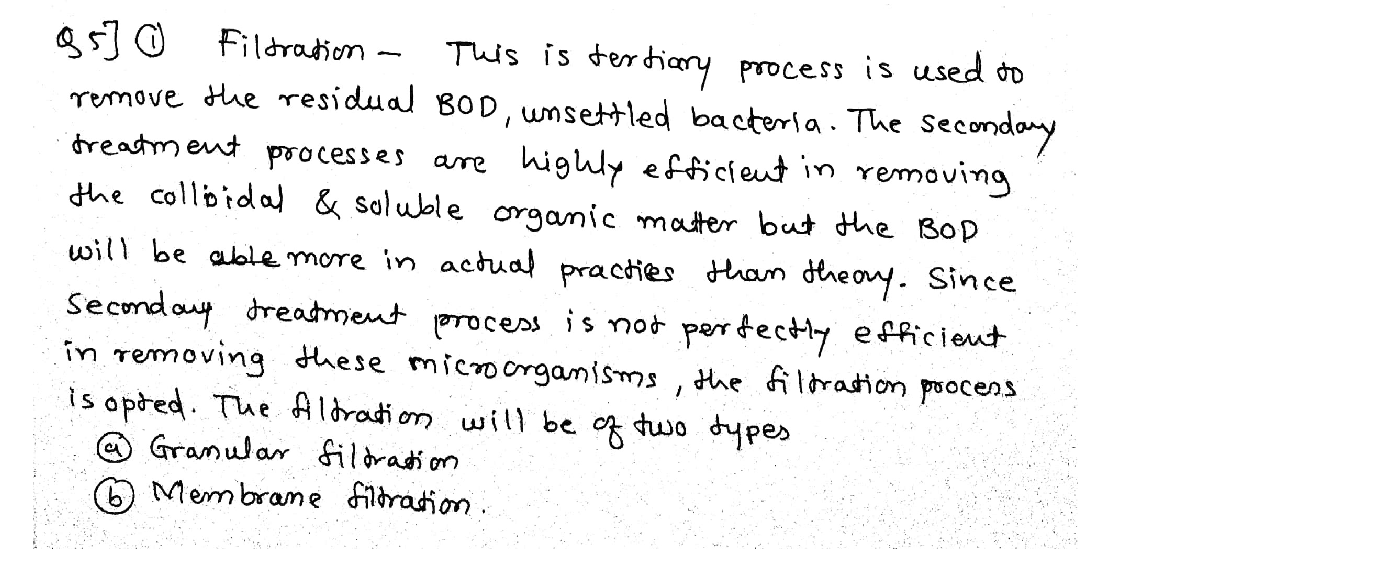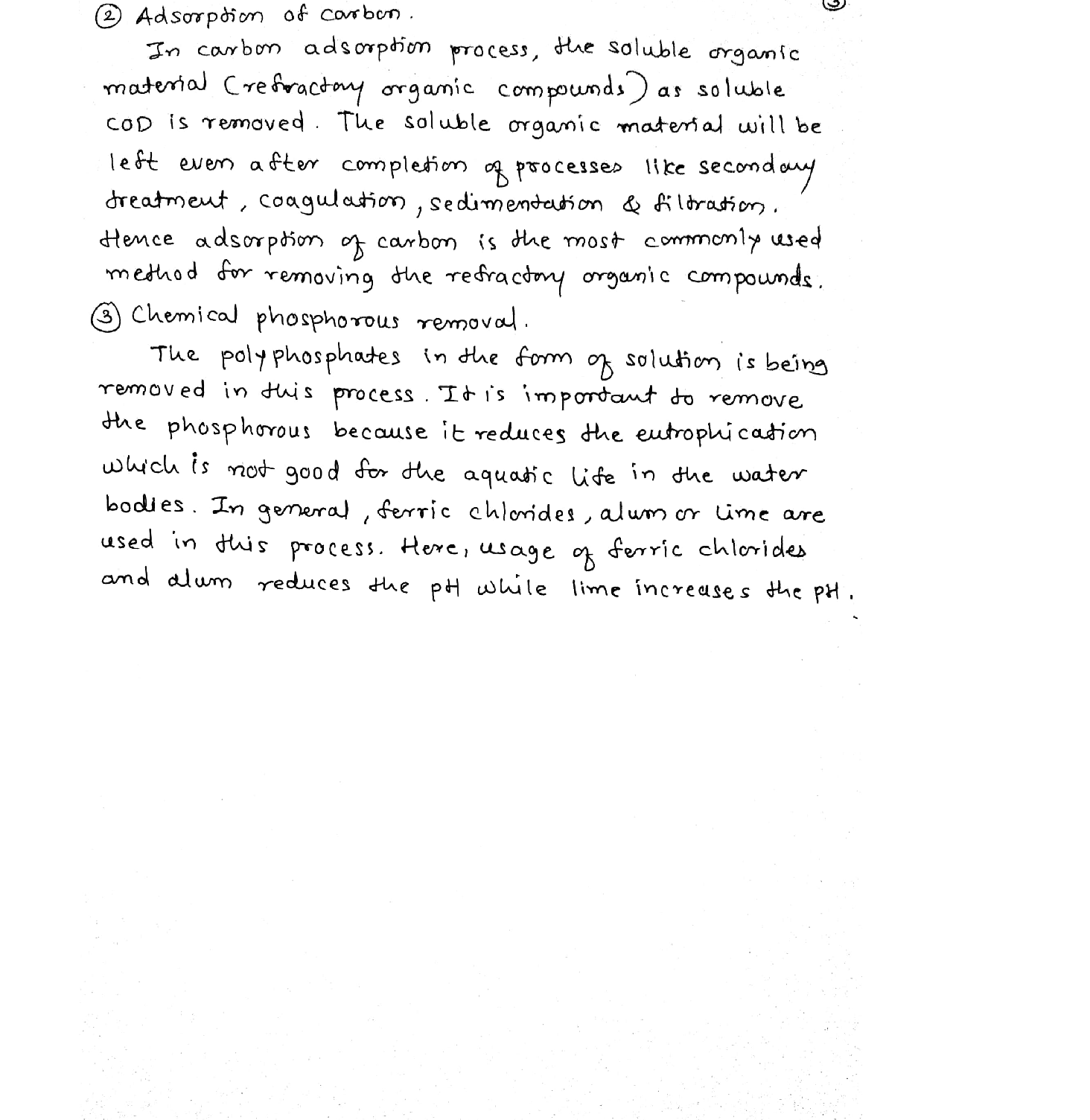Question

Environmental Engineering

• Give three examples of tertial wastewater treatment processes and the pollutants they remove.Verified### Question 39967Environmental Engineering

\mathrm{NH}_{4}^{+}+2 \mathrm{O}_{2} \longrightarrow \mathrm{NO}_{3}^{-}+2 \mathrm{H}^{+}+\mathrm{H}_{2} \mathrm{O}
How many kg of oxygen is required for the complete conversion of 49 kg ofammonia (NH4-N) to nitrate (NO3 -N)? Remember that NH4-N is measured"as N", so you can assume 14 g NH4-N per mole of NH4*.

### Question 39965Environmental Engineering

What is the pH required to decrease the manganeseconcentration in a water supply to 0.01 mg/L if themanganese is in the Mn2+ form?
\mathrm{Mn}(\mathrm{OH})_{2(s)} \leftrightharpoons \mathrm{Mn}^{2+}+2 \mathrm{OH}^{-}
K_{s p}=5 \times 10^{-15}
8.7
6.4
3.8
10.2

### Question 39964Environmental Engineering

\text { What is the equivalent weight of } \mathrm{CaCO}_{3} \text { ? }
50 g/eq
O 20 g/eq
O 100 g/eq
O 12.2 g/eq

### Question 39963Environmental Engineering

Estimate the total weight of sludge in a 200 m anaerobic digester at 45°C with a total solids concentration of 2.0%.Assume a density of 990 kg/m for water, and a specific gravity of 1.25 for the solids portion of the sludge.
194,040 kg
198,000 kg
O 198,827 kg
250,000 kg

### Question 39962Environmental Engineering

Circle any statement(s) that are true. You may select more thanone.
Reaction rate coefficients derived from batch reactor experiments can be applied to any flow-through reactor
If the objective is to allow a chemical reaction to proceed, then plug flow reactors are more efficient than complete mix reactors
A tracer test can be used to determine the hydraulic residence time
If the objective is to allow a chemical reaction to proceed, then-complete mix reactors are more efficient than plug flow reactors

### Question 39961Environmental Engineering

If 160 mg of NaOH are added to 1 m of water with no alkalinity and a starting pH of 7, what would be the approximate final pH?
O 5.4
D 8.6
O 2.4
O 11.6

### Question 39960Environmental Engineering

Considering the redox reactions shown below, which are carried out by Nitrosomonas and Nitrobacter bacteria,respectively, how many moles of O2 are required to completely nitrify one mole of NH4* to NO3?
2 \mathrm{NH}_{4}^{+}+3 \mathrm{O}_{2} \rightarrow 2 \mathrm{NO}_{2}^{-}+4 \mathrm{H}^{+}+2 \mathrm{H}_{2} \mathrm{O}
2 \mathrm{NO}_{2}^{-}+\mathrm{O}_{2} \rightarrow 2 \mathrm{NO}_{3}^{-}
O 2 O 4 O 1.5 03 O 1

### Question 39959Environmental Engineering

Place the unit operations and processes in the most likely sequence for a conventional biological activated sludge wastewater treatment plant with tertiary treatment.

### Question 39958Environmental Engineering

Place the unit operations and processes in the most likely sequence for a conventional surface water treatment plant.
1st unit process in the sequence
2nd unit process in the sequence
3rd unit process in the sequence
4th unit process in the Sequence
5th unit process in the sequence

### Question 39957Environmental Engineering

Circle any statement(s) that are true about the use of alum as a coagulant in a water treatment process. You may select more than one.
It will cause a decrease in the pH of a water with no alkalinity
It will consume the water's alkalinity, if available
It will cause an increase in the pH of a water with no alkalinity
If applied in combination with the correct amount of caustic soda, the pH of the water should not change much

### Submit query